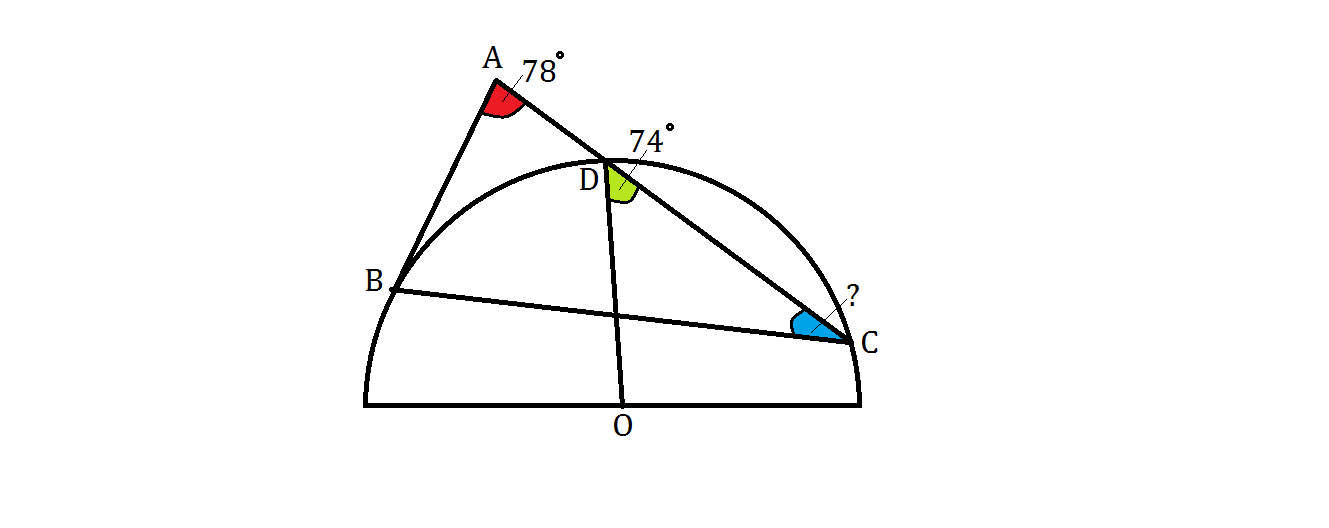# Partially Inscribed TriangleTriangle $ABC$ has vertices $B$ and $C$ on a semicircle centered at $O,$ as shown, with $AB$ tangent to the semicircle at $B$ and $AC$ intersecting the semicircle at point $D.$

If $\angle BAC = 78 ^\circ$(in red) and $\angle ODC = 74 ^\circ$(in green), what is the measure of $\angle BCA$ (in blue) in degrees?

Note: The figure is not drawn to scale.

×